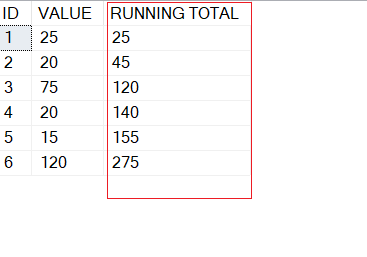Home » How to display Cumulative total in SQL ?

# How to display Cumulative total in SQL ?A cumulative total or running total refers to the sum of values in all cells of a column that precedes the next cell in that particular column.

As you can see the below screenshot which displays a cumulative total in column RUNNING TOTAL for column Value .

Cumulative Total Output Sample:As you can see in above screenshot, the third row of the RUNNING TOTAL column is the sum of all the values of column VALUE in the 1st to the 3rd rows of the ID column, that is 25+ 20+ 75= 120, following similar way the RUNNING TOTAL for the 4th row would be the sum of all the values in VALUE column from the 1st to the 4th rows of the ID, that is 25+ 20+ 75+20 =140. Same technique is followed up to last rows in table.

Lets, prepare a sample table called DataValues,  and insert few records into table.

```CREATE TABLE dbo.DataValues
(ID INT,
[VALUE] INT
)

INSERT INTO  DataValues
(ID, [VALUE])
VALUES
(1, 25),
(2, 20),
(3, 75),
(4, 20),
(5, 15),
(6, 120)

```

Lets implement Query for calculating cumulative/running total.

#### 1. Cumulative Total Using OVER, and ORDER BY CLAUSE

Following Query uses OVER and ORDER BY clause to Calculate Cumulative/Running Total.

```SELECT ID, [VALUE],
SUM([VALUE]) OVER (ORDER BY ID) AS [RUNNING TOTAL]
FROM dbo.DataValues```#### 2. Cumulative Total Using Subquery

Following query uses Subquery to calculate running total.

``` SELECT ID, [VALUE],
(
SELECT SUM(tbl2.[VALUE])
FROM dbo.DataValues AS tbl2
WHERE tbl2.ID <= tbl1.ID
) AS [RUNNING TOTAL]
FROM dbo.DataValues AS tbl1```